The entrance probability theorem shows that if we start from a position say i then the probability of arriving at another position say j for the first time within a time period n is fij(n)

The equation thus becomes:

= P [ Xn = j, Xn-1 ≠ j, Xn-2 ≠ j. … X1 ≠ j| X0 = i]

Now suppose we have Tij =Min {n :Xn = j | X0 = i}:

Thus we see if n=1 the, fij(1)= pij.

Then from the above expressions we get the result:

There is two type of states which we can get from the above result as:

• fii< 1= transient state.
• fii = 1 = recurrent state.

Lastly we come to the need of taking out the average recurrence time i.e. the time taken by the state to return back to its initial position. The equation is:.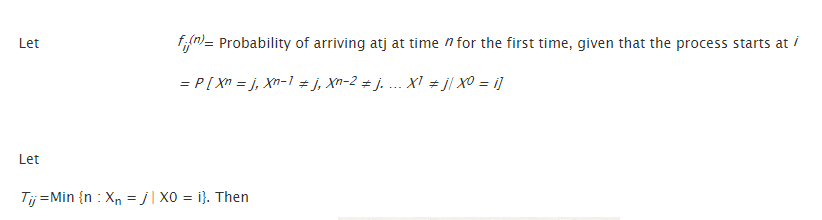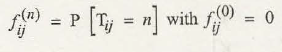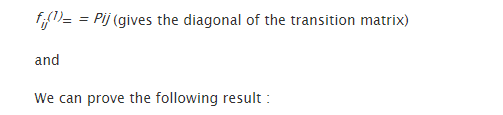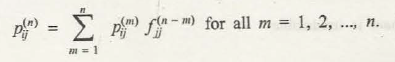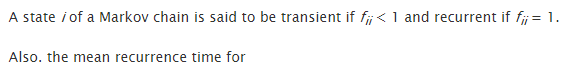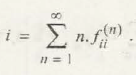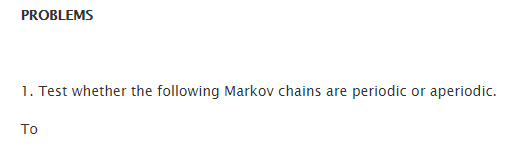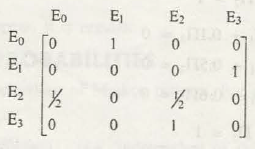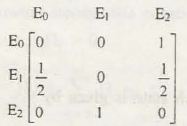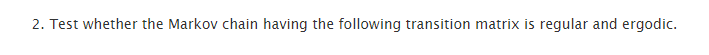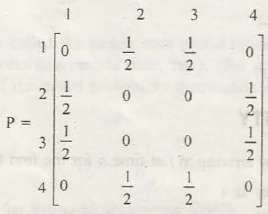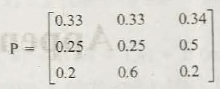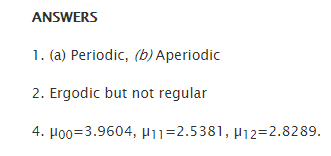### Customer Reviews

My Homework Help
Rated 5.0 out of 5 based on 510 customer reviews at